# Ex.6.4 Q3 Squares and Square Roots - NCERT Maths Class 8

Go back to  'Ex.6.4'

## Question

Find the square root of the following decimal numbers.

(i) $$2.56$$

(ii) $$7.29$$

(iii) $$51.84$$

(iv) $$42.25$$

(v) $$31.36$$

## Text Solution

What is known?

Perfect squares in decimal form

What is unknown?

Square root by using division method

Reasoning:

To find the square root of decimal numbers put bars on the integral part of the number and place bars on the decimal part on every pair of digits starting with the first decimal place.

Steps:

(i)

Square root of $$2.56$$ is calculated as:-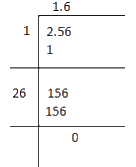$\sqrt {2.56}= 1.6$

(ii)

Square root of $$7.29$$ is calculated as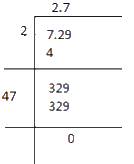$\sqrt {7.29}= 2.7$

(iii)

Square root of $$51.84$$ is calculated as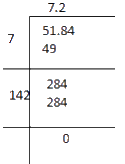$\sqrt {51.84}= 7.2$

(iv)

Square root of $$42.25$$ is calculated as follows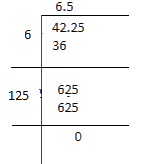$\sqrt {42.25}= 6.25$

(v)

Square root of $$31.36$$ is calculated as follows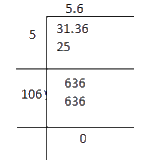$\sqrt {31.36}= 5.6$

Learn from the best math teachers and top your exams

• Live one on one classroom and doubt clearing
• Practice worksheets in and after class for conceptual clarity
• Personalized curriculum to keep up with school